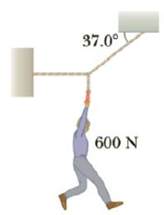Chapter 4, Problem 35P

Chapter
Section
Textbook Problem

(a) Find the tension in each cable supporting the 6.00 × 102-N cat burglar in Figure P4.35. (b) Suppose the horizontal cable were reattached higher up on the wall. Would the tension in the other cables increase, decrease, or stay the same? Why?Figure P4.35

(a)

To determine
The tension in each of the cables.

Explanation

Section 1:

To determine: The tension in the left cable.

Answer: The tension in the right cable is 996.68 N.

Explanation:

Given Info: Weight of the cat burglar is 6.00×102N .

The free body diagram is given below.

From the diagram,

T2sin37ο=W                     (I)

• T1 and T2 are the tensions in the left and right wires respectively.
• W is the weight of the cat burglar.

Substitute 6.00×102N for W in Equation (I) to get T2 .

T2=6.00×102N0.602=996.68N

The tension in the right cable is 996

(b)

To determine
The tension in the right cable.

Still sussing out bartleby?

Check out a sample textbook solution.

See a sample solution

The Solution to Your Study Problems

Bartleby provides explanations to thousands of textbook problems written by our experts, many with advanced degrees!

Get Started

Lysine does not cure herpes infections. T F

Nutrition: Concepts and Controversies - Standalone book (MindTap Course List)

Complete the following table:

Chemistry & Chemical Reactivity

How can astronomers detect neutrinos from the sun?

Horizons: Exploring the Universe (MindTap Course List)